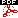missrc MLEs for incomplete n-way contingency tables missrc

# SAS Macro Programs: missrc

\$Version: 1.4 (6 Nov 2001)
Michael Friendly
York University

## The missrc macro (get missrc.sas)

### MLEs for incomplete n-way contingency tables

The missrc macro estimates cell probabilities in an n-way table with ignorable missing data (missing completely at random [MCAR], or missing at random [MAR]) on the table variables. For example, in a longitudinal survey, some respondents may have missing responses on one or more occasions, and it is desired to estimate the cell probabilities using the available information. The results are equivalent to the use of the EM algorithm.

### Method

Lipsitz etal (1998) show that in this case the cell probabilities may be estimated as a specially constructed Poisson generalized linear model with a structured design matrix and an offset containing various marginal totals. The equivalent Poisson GLM is
```
E(f)  =  X p  + g

```
where f is a vector containing the cell frequencies, X is the design matrix, p is the vector of cell probabilities, and g is a vector of marginal totals used as an offset variable. Friendly (1999)generalizes their method to n-way tables.

The MISSRC macro constructs this design matrix and offset variable. It then estimates the cell probabilities using PROC GENMOD, and returns a table with the estimates, their standard errors, and fitted cell frequencies.

## Usage

The missrc macro is called with keyword parameters. Only the VAR= parameters is required. The arguments may be listed within parentheses in any order, separated by commas. For example:
``` %missc(var=R C, count=count);
```

### Parameters

Default values are shown after the name of each parameter.
DATA=_LAST_
Specifies the name of the input data set to be analyzed. If omitted, the most recently created data set is used.
VAR=
Specifies the names of the table variables. In this version, all VAR= variables must be numeric, with non-negative integer levels. The missing level must have the SAS missing value, .
COUNT=COUNT
Specifies the name of the variable holding the cell frequencies. If not specified, COUNT=COUNT is assumed.
OUT=CELLS
Specifies the name of the output data set containing estimated cell probabilities, standard errors, etc.
DESIGN=DESIGN
Specifies the name of the output design matrix data set

### Example

Little & Rubin (1987, p. 183) gave the following table:
```                    |  C1     C2   Missing
--------+---------------------
R1      | 100     50     30
R2      |  75     75     60
Missing |  28     60
```
Create a SAS data set, as follows. The frequency variable is named COUNT, the default for MISSRC. Note that missing values for R and C are specified as .
```data little;
input R C count @@;
cards;
1  1  100    1  2  50    1  .  30
2  1   75    2  2  75    2  .  60
.  1   28    .  2  60
;
%include macros(missrc);        *-- or include in an autocall library;
%missrc(data=little, var=R C);
```
The following output data set is produced. The variable P is the observed cell probability, ESTIMATE is the MLE, and FITTED is the estimated cell frequency.
```  R    C    COUNT       P       PARM    ESTIMATE     STDERR      FITTED

.    1      28      .                   .          .             .
.    2      60      .                   .          .             .
1    .      30      .                   .          .             .
1    1     100     0.33333    P11      0.27947    0.022310    133.589
1    2      50     0.16667    P12      0.17402    0.020978     83.184
2    .      60      .                   .          .             .
2    1      75     0.25000    P21      0.23872    0.022660    114.108
2    2      75     0.25000    P22*     0.30778    0.025298    147.120
```

### See also

powerrxc Power analysis for Chi-sqare tests of independence

### References

Friendly, M. (1999). Note on ``Obtaining the maximum likelihood estimates in incomplete R x C Contingency tables...'', J. Computational. and Graphical Statistics, 8, in press.

Lipsitz, S. R., Parzen, M. and Molenberghs, G. (1998). ``Obtaining the maximum likelihood estimates in incomplete R x C Contingency tables using a Poisson generalized linear model''. J. Computational. and Graphical Statistics, 7, 356--376.